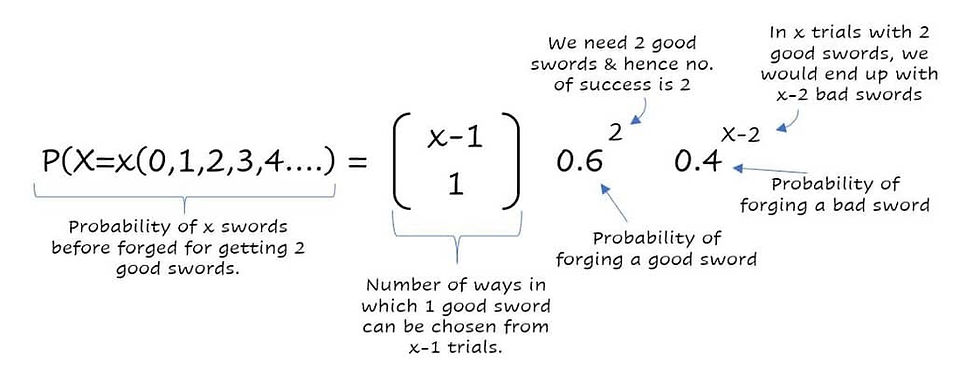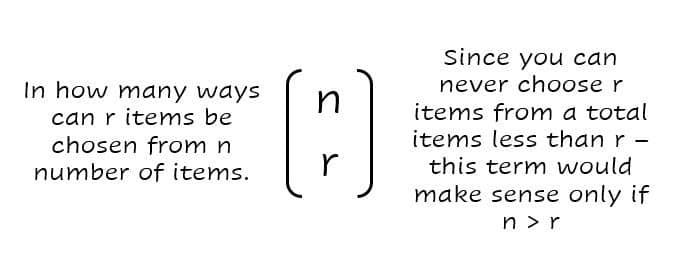top of page
Search
• Bharath Reddy

# Negative Binomial Distribution

PROBABILITY DISTRIBUTIONS EVERYONE SHOULD KNOW INTUITIVELY

Sword maker and 2 good swords.Why should you know this distribution?

Between the beginnings of the time and the First Ages - aided by a “Nazgul” (fire breathing dragon), the peace of middle earth was under threat from constant attacks by the dark lord. Nazgul was feared across the realms and you are the lord protector of these realms and have been called to put an end to Nazgul.

Nazgul has two hearts filled with fire and can be killed only by a direct stab to its heart. Only a sword forged by the hobbits can pierce its armor and reach its heart and even then the fire in the heart would melt the sword. So you would need two of these swords in your quest.

Sword making is an art that is unknown beyond the hobbit race. To craft a Nazgul killing sword, a hobbit would have to forge multiple swords and would have a 6 in 10 chance of making a sword good enough to kill the Nazgul.

Consider again the case of Binomial distribution I discussed earlier, here we have a hobbit who wants to produce two good swords using a forge with a probability of 60% of making a good sword in each attempt. Under Binomial distribution, we answer questions like “if our hobbit forges five swords, what is the probability of getting at least two good swords.” Here though, if only two good swords are needed, the hobbit would forge swords one by one and stop when he has two good swords for you.

You would need to understand Negative Binomial distribution to answer questions like

“Given the hobbit can forge a good sword only 6 of 10 times — how many swords does he have to forge before he can give us two good ones.”

What is the probability that hobbit would forge exactly 5 swords to be able to give us 2 good ones?

Suppose you have time only to forge 4 swords, how confident can the hobbit be that he would have two good ones within the available time?

If you want to be at least 95% confident, at least how many swords you would want the hobbit to forge?

In general, when-ever the probability of success is held constant and you want to know the number of trials it would take to get a particular number of successes you would use a Negative Binomial Distribution.
Okay, I’m interested — tell me how this works?

Ok so our good hobbit gets to the task and starts forging the swords, He forges the first sword, the second one and continues. Somewhere down the line, he manages to forge a sword good enough to take out one heart of the Nazgul. The task is not done yet! Remember — his aim is to give you two good swords. So he continues in his forging process until the second good sword is forged.

Let’s say he forges a total of x swords in his quest to give you 2 good swords. For good two swords, we know that the very last forged sword must be a good one, otherwise, he would have already had the desired number of good swords, and we would have stopped right there.The last sword being a success, the ﬁrst x -1 forged swords should have had the first good sword. So lets put down the information we know as of now:

• Total no. of success desired / no. of good swords: 2 (denoted by S below)

• Total no. of trials made: x

• The Xth trial was a good sword.

• We know the previous x-1 trials should have had the 1st good sword.

Now if you remember getting (s-1) success, from (x-1) trials becomes a binomial distribution with equationThe first figure in parenthesis gives the number of ways in which (s-1) successes aka. a good sword can be selected from (x-1) trials. In our case, S is 2 and therefore s-1 becomes 1

Substituting the above two our equation now becomesBy substituting various values of x we can find corresponding probabilities of x. Now we can answer all the questions we had in our minds.

NOTE: At this point, if you still are not conceptually clear please read my article on the binomial distribution

1. What is the probability that hobbit would forge exactly 5 swords to be able to give us 2 good ones?

Plugin 5 instead of x in the above equation and you would get 0.092 ie. 9.2% chance of our hobbit giving us 2 good swords if he forges exactly 5 swords.2. Suppose you have time only to forge 4 swords, how confident can the hobbit be that he would have two good ones within the available time?

Let me rephrase this question to be able to understand it better. The question is, how confident can you be, to have 2 good swords if the hobbit had time only to forge 4 swords. In other words the probability of needing at most four trials to give 2 good swords.

Note here at most means can be, 0 trials, 1 trial, 2 trials, 3 trials or 4 trials. You would replace x by 0,1,2,3 and 4 and add all the outputs and get your probability.You will see that the first term would make sense only for x = 2 onwards. We would see that the probability of needing at most 4 trials is 0.8208 and hence you can be about 82% confident.

3. If you want to be at least 95% confident, at least how many swords you would want the hobbit to forge?

If we were to draw a table of x vs P(x) i.e. what is the probability for each value of x we have something like below. I have put this together using simple excel and explained the formula for you to recreate it for your usage.Looking at the above template in the “at most x” column, we infer that we should be prepared for at least 6 trials since five trials yield only 91.30% confidence and 6 trials yield 95.90% confidence.

Conclusion
People with a grasp of probability distributions have an additional quality set of tools at hand when evaluating their choices, assessing risk, and avoiding the unpleasant results than can accrue from insufficient preparation. When you understand these distributions and its often surprising results, you’ll be well ahead of the masses.

Happy Learning.